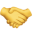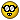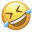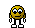What's new
Fantasy Football - Footballguys Forums

Welcome to Our Forums. Once you've registered and logged in, you're primed to talk football, among other topics, with the sharpest and most experienced fantasy players on the internet.

# Math question (1 Viewer)

#### the rover

##### Footballguy
Yesterday I was playing pull tabs at a local bar. The game had 6000 tickets (assume none had been played). There are 15 tickets that are scratch offs, so you can win the main prizes. I put \$100 in and got 6 of the scratch offs on the first pull. What were the odds?

Nobody likes math

I’m sure I could figure this out but I’d bet someone like @Ignoratio Elenchi will do it

Nobody likes math

I’m sure I could figure this out but I’d bet someone like @Ignoratio Elenchi will do it
I like math, but not gambling. No idea what constitutes a “pull tab”.

•jhib
How many tickets did you pull?

Pull my finger.

50/50

•Leroy Hoard
To calculate the odds of getting 6 out of the 15 winners in a drawing with 6000 entries, we need to use combinatorics.

The total number of ways to choose 6 winners out of the 15 available winners is given by the combination formula:

C(n, r) = n! / (r!(n-r)!),

where n is the total number of items and r is the number of items to be chosen.

In this case, n = 15 (total number of winners) and r = 6 (number of winners to be chosen).

C(15, 6) = 15! / (6!(15-6)!) = 5005.

So, there are 5005 possible combinations of choosing 6 winners out of the 15.

Now, let's calculate the odds. Odds are typically expressed as the ratio of the number of successful outcomes to the number of unsuccessful outcomes.

The number of successful outcomes is 1 because you want to calculate the odds of getting exactly 6 winners.

The number of unsuccessful outcomes is the total number of combinations minus the number of successful outcomes:

Unsuccessful outcomes = Total combinations - Successful outcomes
= 5005 - 1
= 5004.

Therefore, the odds of getting 6 out of the 15 winners in a drawing with 6000 entries are 1:5004.

•Dan Lambskin••Steeler, fatness, mr. furley and 2 others
To calculate the odds of getting 6 out of the 15 winners in a drawing with 6000 entries, we need to use combinatorics.

The total number of ways to choose 6 winners out of the 15 available winners is given by the combination formula:

C(n, r) = n! / (r!(n-r)!),

where n is the total number of items and r is the number of items to be chosen.

In this case, n = 15 (total number of winners) and r = 6 (number of winners to be chosen).

C(15, 6) = 15! / (6!(15-6)!) = 5005.

So, there are 5005 possible combinations of choosing 6 winners out of the 15.

Now, let's calculate the odds. Odds are typically expressed as the ratio of the number of successful outcomes to the number of unsuccessful outcomes.

The number of successful outcomes is 1 because you want to calculate the odds of getting exactly 6 winners.

The number of unsuccessful outcomes is the total number of combinations minus the number of successful outcomes:

Unsuccessful outcomes = Total combinations - Successful outcomes
= 5005 - 1
= 5004.

Therefore, the odds of getting 6 out of the 15 winners in a drawing with 6000 entries are 1:5004.

Could be complete ******** but I’m inclined to believe it.

•dutch and mr. furley
•The Z Machine
To calculate the odds of getting 6 out of the 15 winners in a drawing with 6000 entries, we need to use combinatorics.

The total number of ways to choose 6 winners out of the 15 available winners is given by the combination formula:

C(n, r) = n! / (r!(n-r)!),

where n is the total number of items and r is the number of items to be chosen.

In this case, n = 15 (total number of winners) and r = 6 (number of winners to be chosen).

C(15, 6) = 15! / (6!(15-6)!) = 5005.

So, there are 5005 possible combinations of choosing 6 winners out of the 15.

Now, let's calculate the odds. Odds are typically expressed as the ratio of the number of successful outcomes to the number of unsuccessful outcomes.

The number of successful outcomes is 1 because you want to calculate the odds of getting exactly 6 winners.

The number of unsuccessful outcomes is the total number of combinations minus the number of successful outcomes:

Unsuccessful outcomes = Total combinations - Successful outcomes
= 5005 - 1
= 5004.

Therefore, the odds of getting 6 out of the 15 winners in a drawing with 6000 entries are 1:5004.
no. the odds of getting 1 is 6000:1. there is no way that getting 6 is only 5000:1

•fatness
How many tickets did you pull?
100
C(15, 6) / C(6000, 100)

ChatGPT says the answer 1 in 3042.•mr. furley
It's 1 in 12491473 or .00000800546%

I got that from a formula online. It's a non-replacement probability issue.

It's a combinatorics problem. You're drawing 100 out of 6000 and you need to pull 6 distinct pull tab tickets out of a total of 15 distinct pull tabs.

And your odds of getting a pull tab with one ticket pulled is actually one in four hundred. I think.

This is the website.

I'm just guessing, by the way. But I'd go to bat for it.

To calculate the odds of getting 6 out of the 15 winners in a drawing with 6000 entries, we need to use combinatorics.

The total number of ways to choose 6 winners out of the 15 available winners is given by the combination formula:

C(n, r) = n! / (r!(n-r)!),

where n is the total number of items and r is the number of items to be chosen.

In this case, n = 15 (total number of winners) and r = 6 (number of winners to be chosen).

C(15, 6) = 15! / (6!(15-6)!) = 5005.

So, there are 5005 possible combinations of choosing 6 winners out of the 15.

Now, let's calculate the odds. Odds are typically expressed as the ratio of the number of successful outcomes to the number of unsuccessful outcomes.

The number of successful outcomes is 1 because you want to calculate the odds of getting exactly 6 winners.

The number of unsuccessful outcomes is the total number of combinations minus the number of successful outcomes:

Unsuccessful outcomes = Total combinations - Successful outcomes
= 5005 - 1
= 5004.

Therefore, the odds of getting 6 out of the 15 winners in a drawing with 6000 entries are 1:5004.
no. the odds of getting 1 is 6000:1. there is no way that getting 6 is only 5000:1
I think the odds of getting 1 of 15 winners in a pool of 6000 tickets is better than 6000:1. Especially if you’re drawing 100 tickets.

I'm just guessing, by the way. But I'd go to bat for it.
C(15, 6) / C(6000, 100)

I asked ChatGPT to give me the answer to the combinatorial above, which mathematically describes what you posted, I think.

But I get the same result as you, using your link. I’d trust that one.

And your odds of getting a pull tab with one ticket pulled is actually one in four hundred. I think.
Seems right. 15 out of 6000 is the same as 1 in 400.

•fatness
To calculate the odds of getting 6 out of the 15 winners in a drawing with 6000 entries, we need to use combinatorics.

The total number of ways to choose 6 winners out of the 15 available winners is given by the combination formula:

C(n, r) = n! / (r!(n-r)!),

where n is the total number of items and r is the number of items to be chosen.

In this case, n = 15 (total number of winners) and r = 6 (number of winners to be chosen).

C(15, 6) = 15! / (6!(15-6)!) = 5005.

So, there are 5005 possible combinations of choosing 6 winners out of the 15.

Now, let's calculate the odds. Odds are typically expressed as the ratio of the number of successful outcomes to the number of unsuccessful outcomes.

The number of successful outcomes is 1 because you want to calculate the odds of getting exactly 6 winners.

The number of unsuccessful outcomes is the total number of combinations minus the number of successful outcomes:

Unsuccessful outcomes = Total combinations - Successful outcomes
= 5005 - 1
= 5004.

Therefore, the odds of getting 6 out of the 15 winners in a drawing with 6000 entries are 1:5004.
no. the odds of getting 1 is 6000:1. there is no way that getting 6 is only 5000:1
Idk that's what chatgpt gave and it's not always right.

Yesterday I was playing pull tabs at a local bar. The game had 6000 tickets (assume none had been played). There are 15 tickets that are scratch offs, so you can win the main prizes. I put \$100 in and got 6 of the scratch offs on the first pull. What were the odds?
6000/100
15:6 or like Chicago says 6 to 4

1 in a Million if you ask me

Last edited:
You also need to ask are you interested in getting exactly 6 or at least 6

•Alex P Keaton

Replies
119
Views
2K
Replies
14
Views
692
Replies
39
Views
689